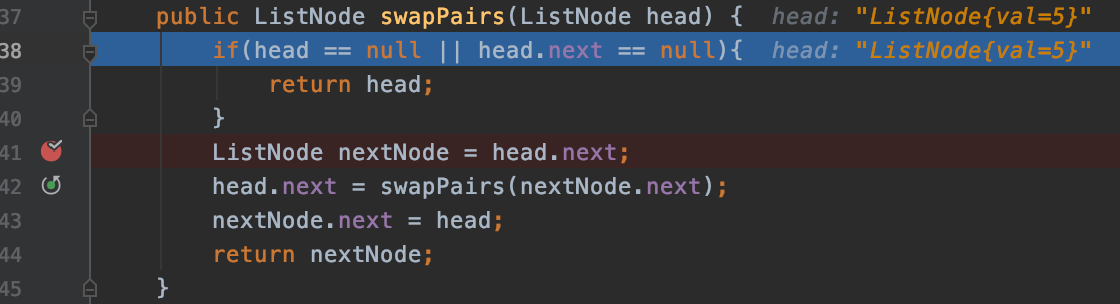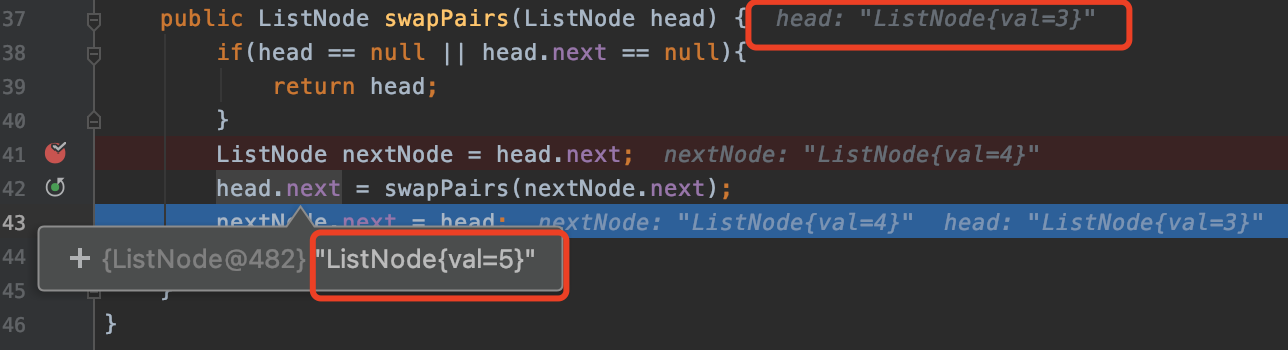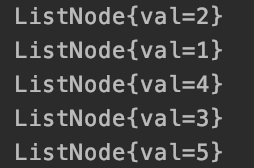# 链表算法题之中等级别，debug调试更简单

#### 文章简述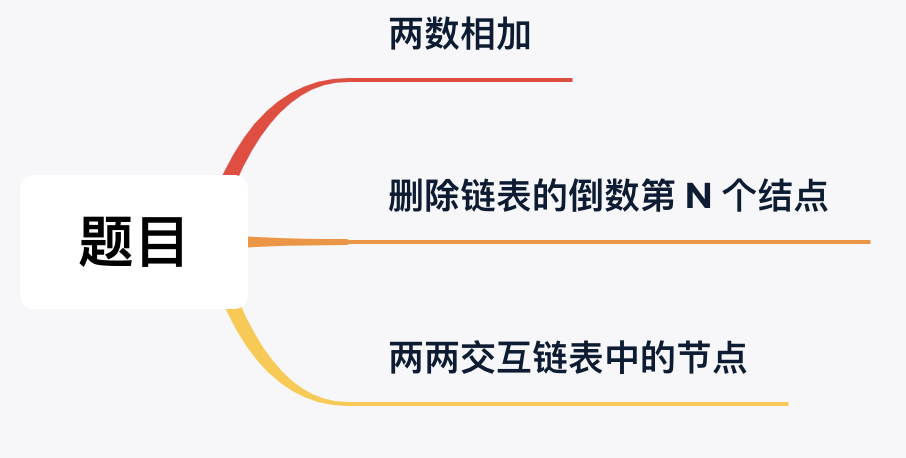#### 一，两数相加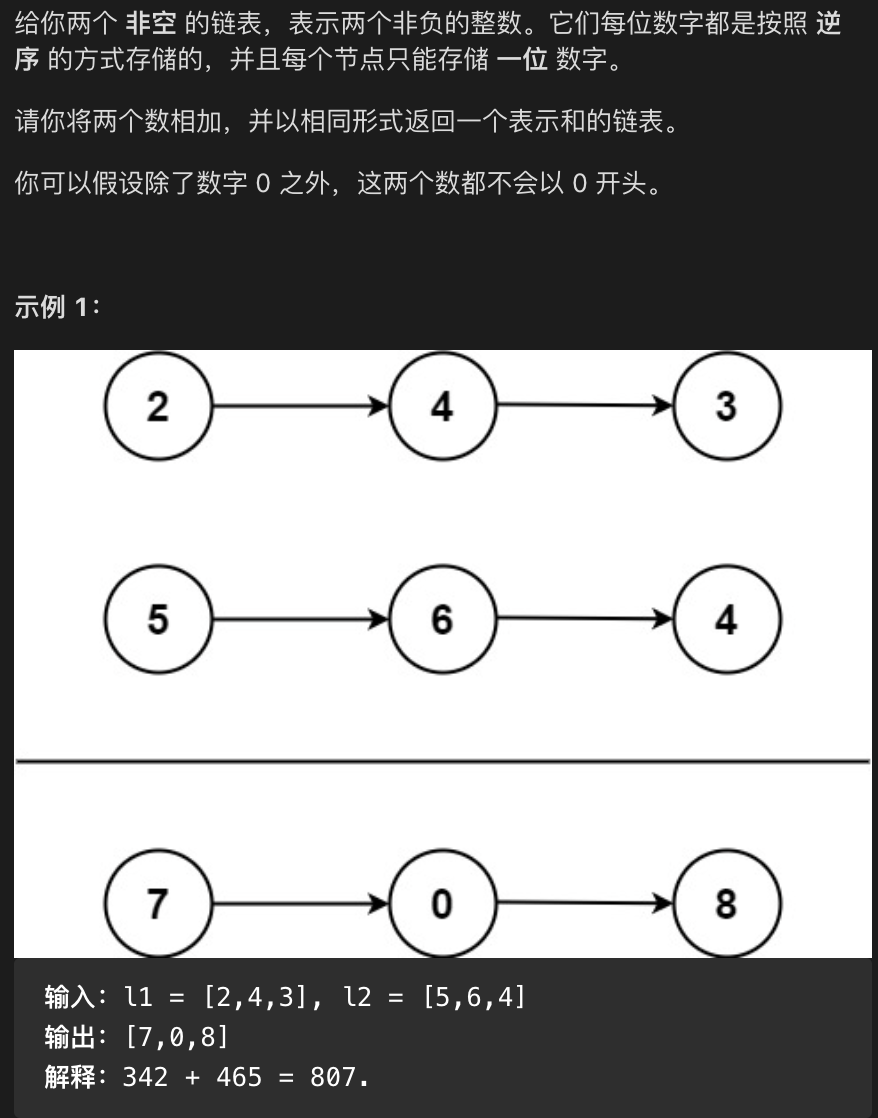##### 1.1 题目分析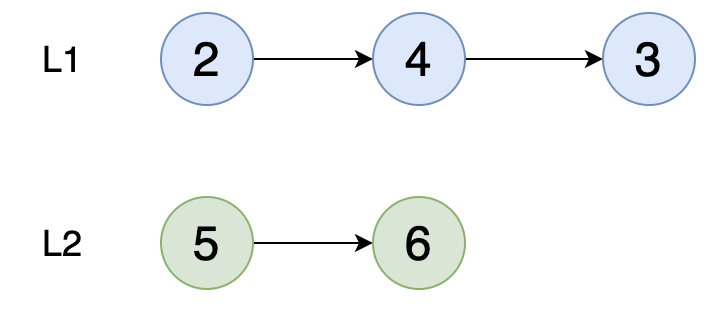L2 链表少了一个节点，像这种情况我们就需要在高位用【0】进行补位。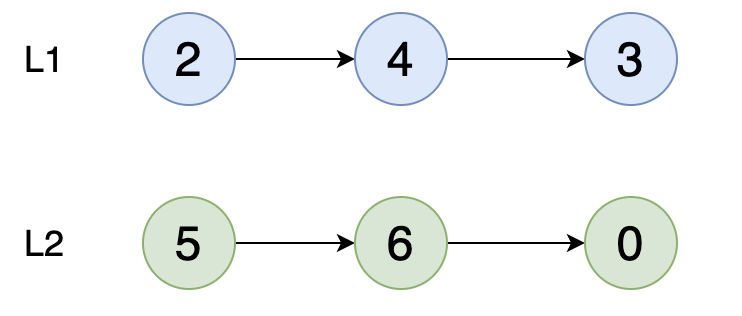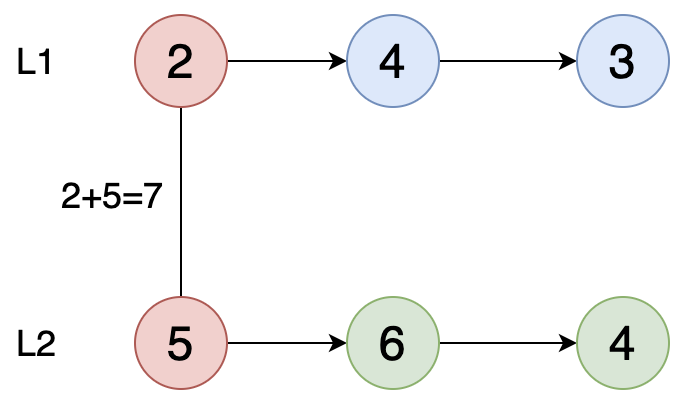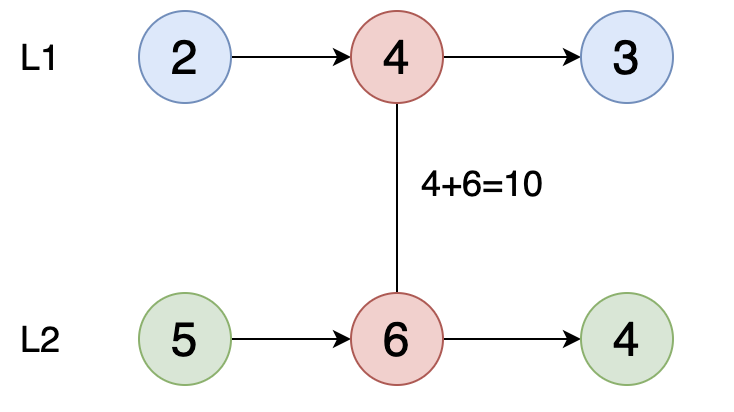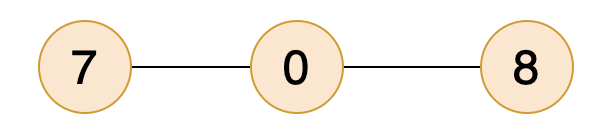##### 1.2 代码分析

``````	// 创建链表-L1
ListNode l1 = new ListNode(2);
ListNode l2 = new ListNode(4);
ListNode l3 = new ListNode(3);

ListNodeFun listNodeFun = new ListNodeFun();
listNodeFun.add(l1);
listNodeFun.add(l2);
ListNode listNode1 = listNodeFun.add(l3);

ListNodeFun listNodeFun2 = new ListNodeFun();
// 创建链表-L2
ListNode l11 = new ListNode(5);
ListNode l22 = new ListNode(6);
ListNode l33 = new ListNode(4);
listNodeFun2.add(l11);
listNodeFun2.add(l22);
ListNode listNode2 = listNodeFun2.add(l33);
``````

``````    if(null == l1 || null == l2){
return null;
}
// 初始化头指针
ListNode head = new ListNode();
ListNode cur = head;
// 定义变量保存进位值
int temp = 0;
while(null != l1 || null != l2){
// 获取每个节点的值
int x = l1 == null ? 0 : l1.val;
int y = l2 == null ? 0 : l2.val;
// 两数相加
int sum = x + y + temp;
// 获取相加结果
temp = sum  / 10;
// 获取低位（个位）
sum = sum % 10;
// 创建新的节点
cur.next = new ListNode(sum);
// 移动指针
cur = cur.next;
// 移动链表指针，要判断为空，否则会空针
if(null != l1){
l1 = l1.next;
}
if(null != l2){
l2 = l2.next;
}
}
if(1 == temp){
cur.next = new ListNode(1);
}
return head.next;
}
``````
##### 1.3 debug 调试
###### 第一步，2+5

【7】不需要进位，创建新的链表进行保存。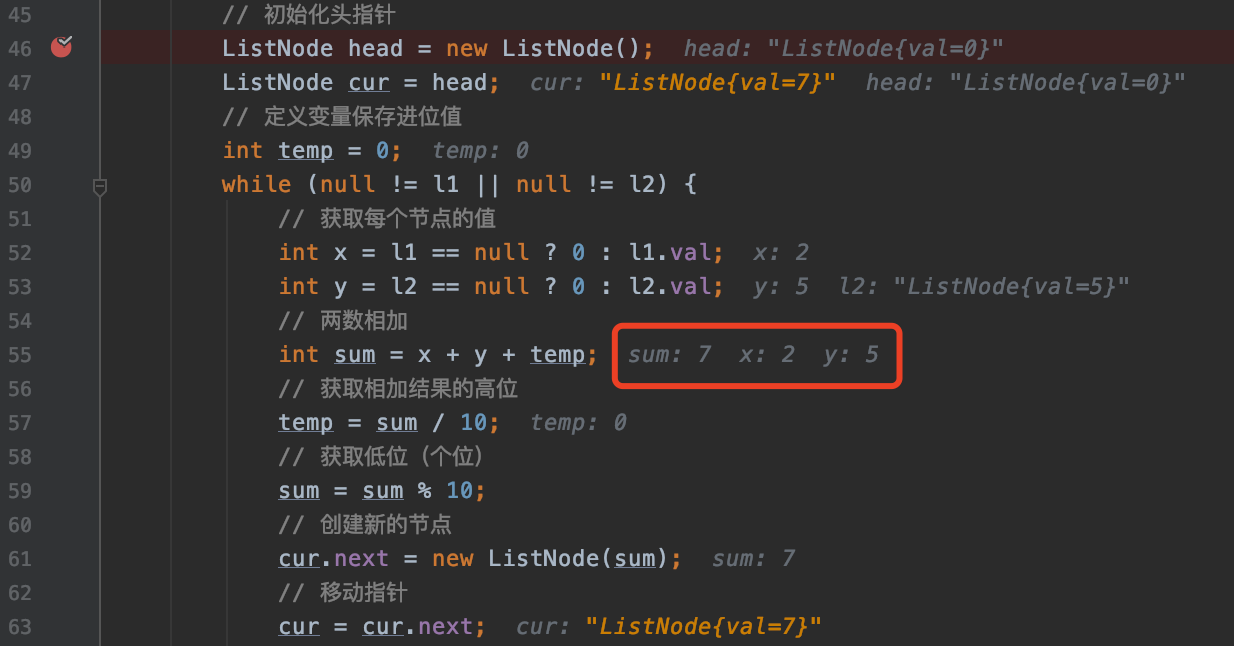###### 第二步，4+6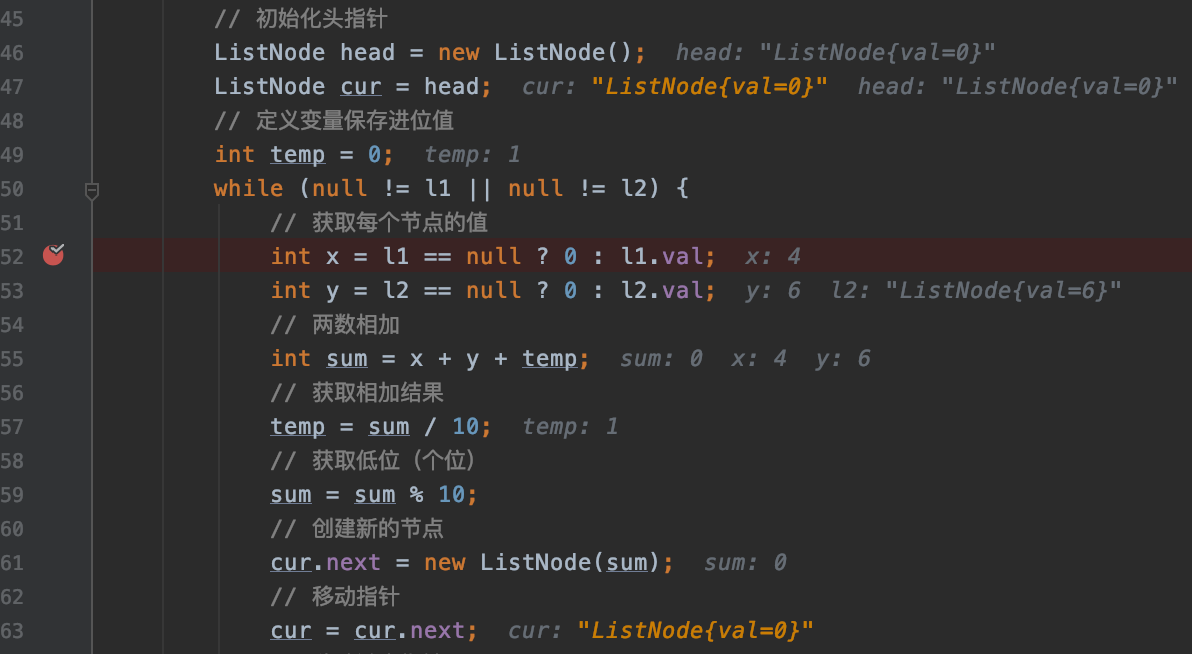###### 第三步，3+4+1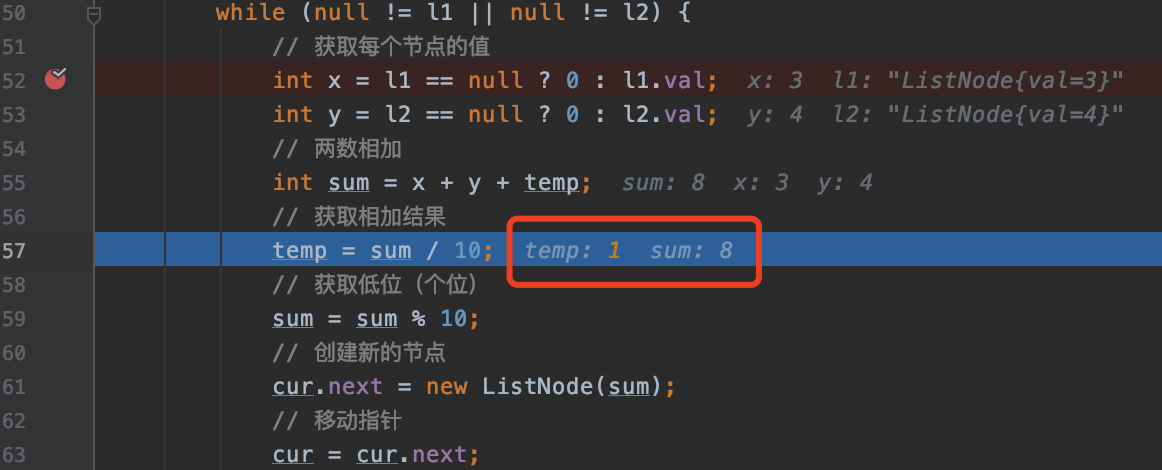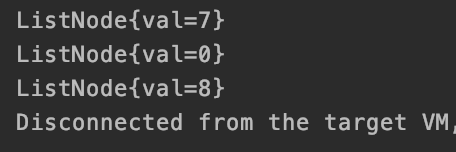#### 二，删除链表的倒数第 N 个结点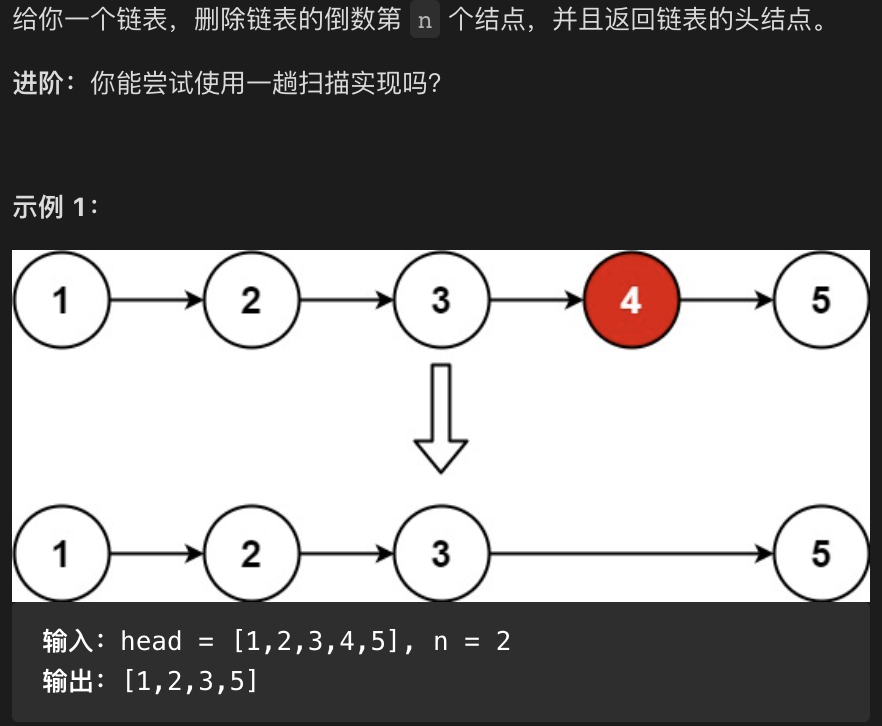##### 2.1 题目分析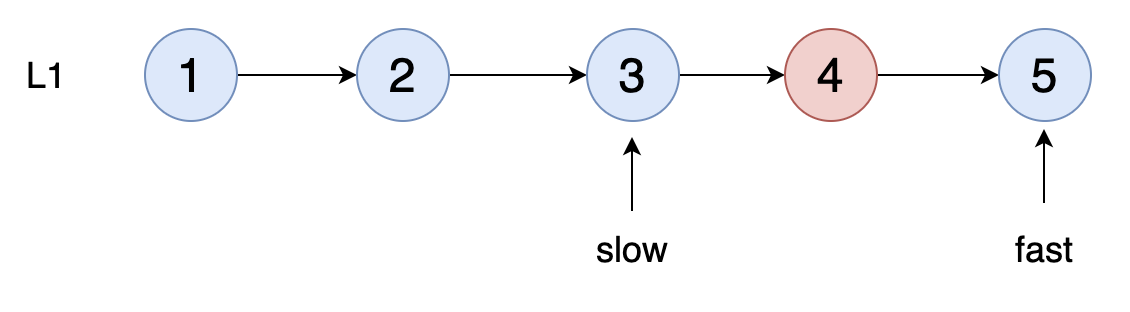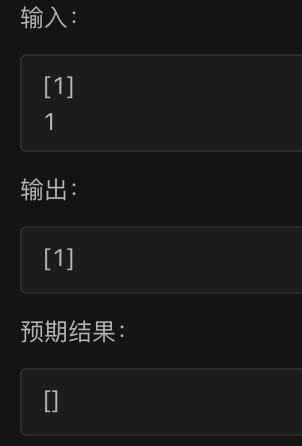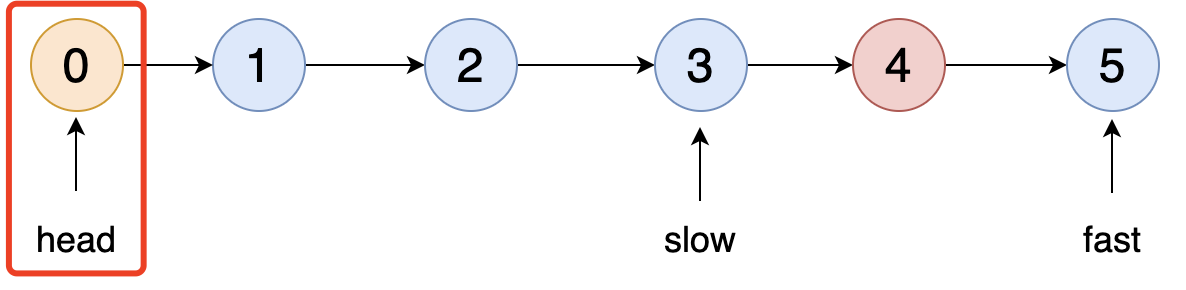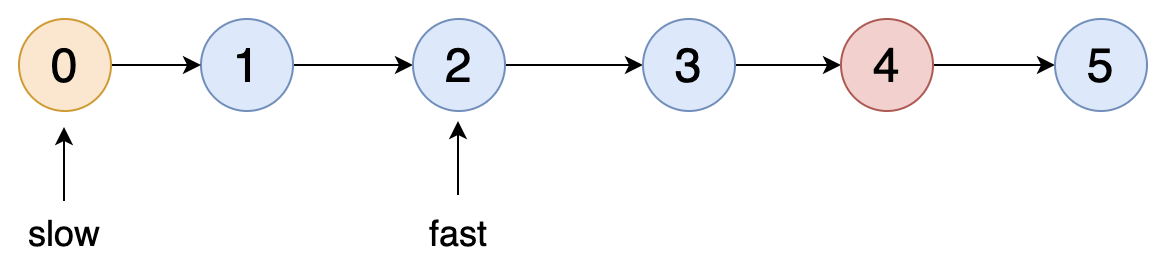##### 2.2 代码分析

``````public ListNode removeNthFromEnd(ListNode head, int n) {
ListNode pre = new ListNode();
pre.next = head;
// 定义双指针
ListNode slow = pre;
ListNode fast = head;
// 先将快指针移动n个节点
while(--n>0){
fast = fast.next;
}
while(fast.next != null){
fast = fast.next;
slow = slow.next;
}
slow.next = slow.next.next;
return pre.next;
}
``````
##### 2.3 debug 调试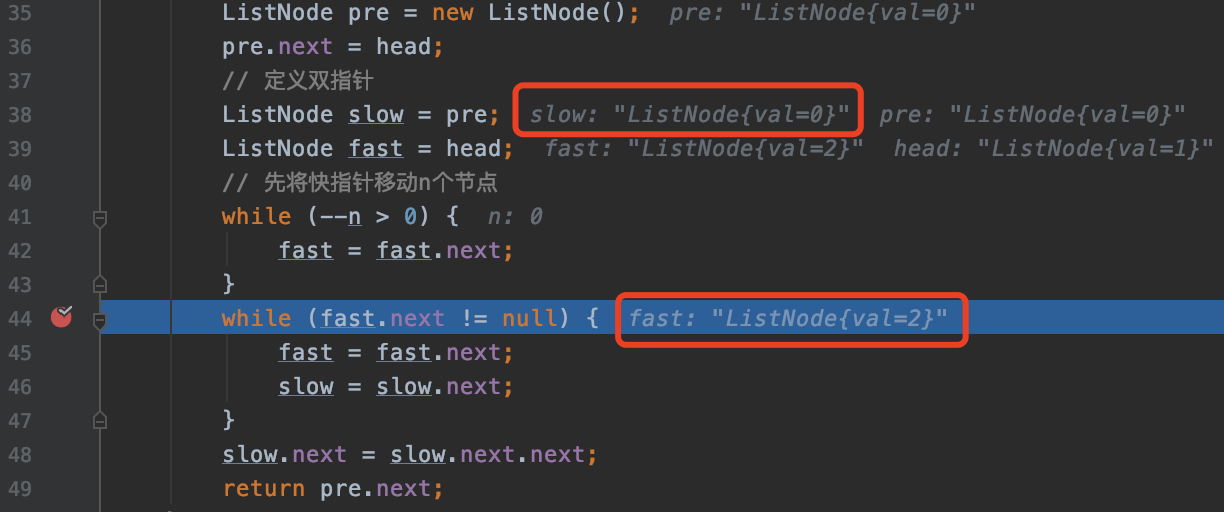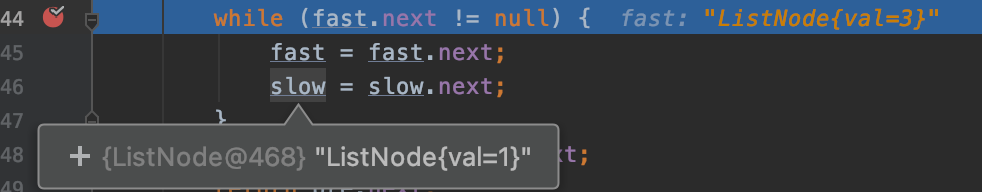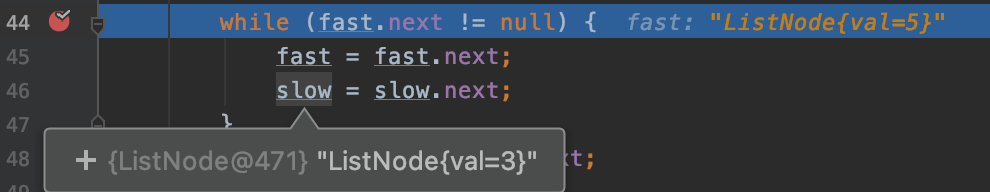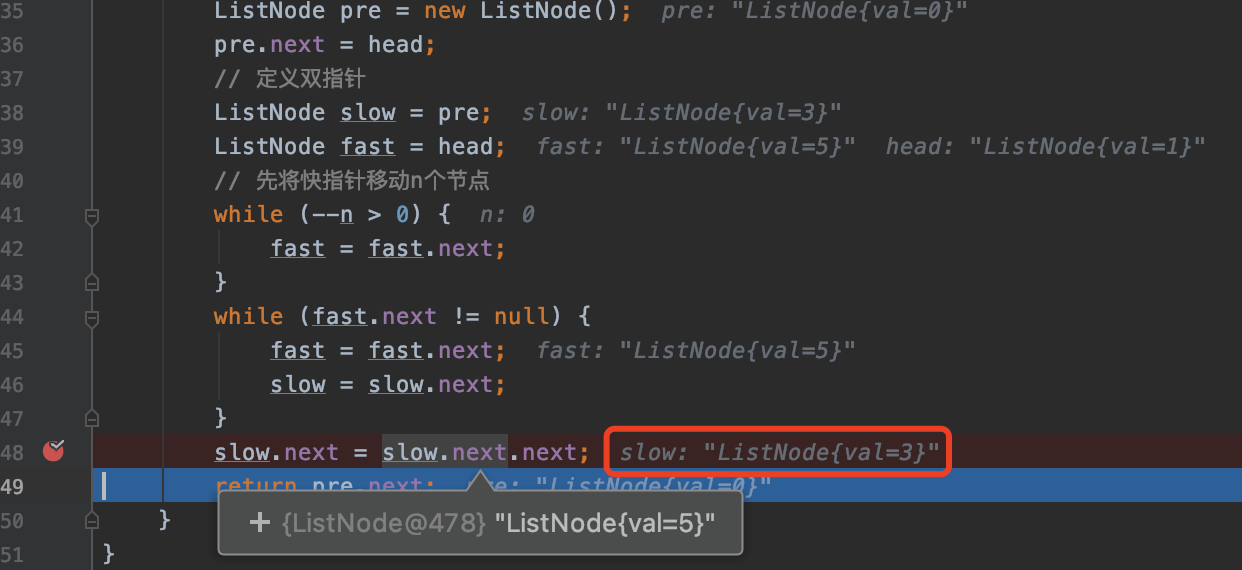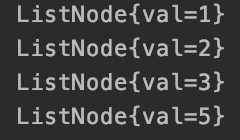#### 三，两两交互链表中的节点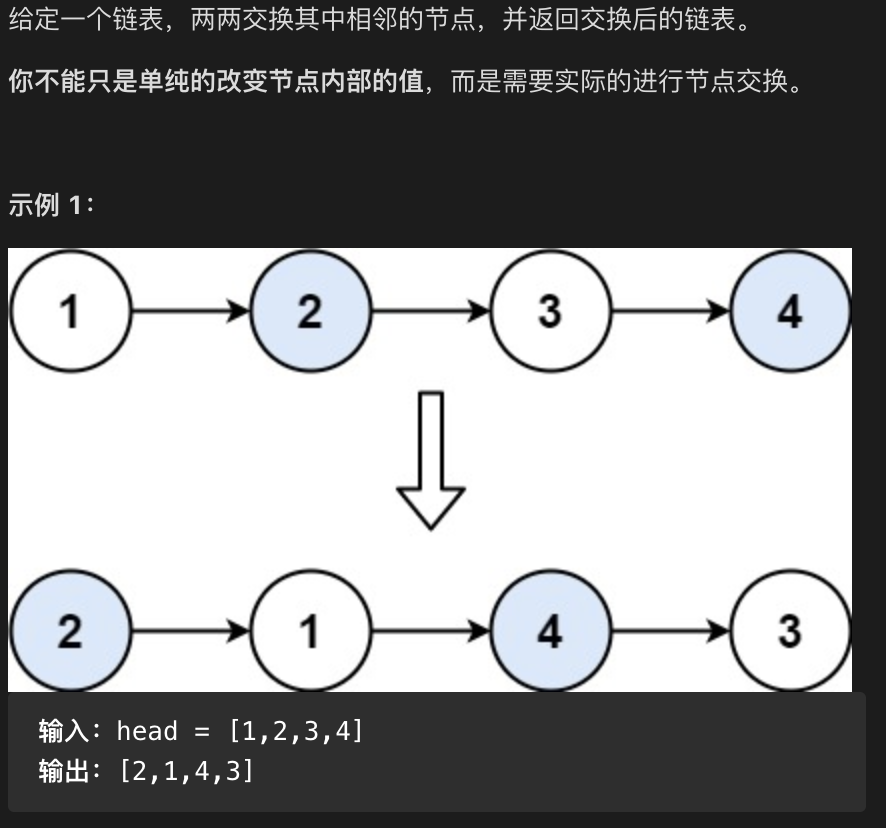##### 3.1 题目分析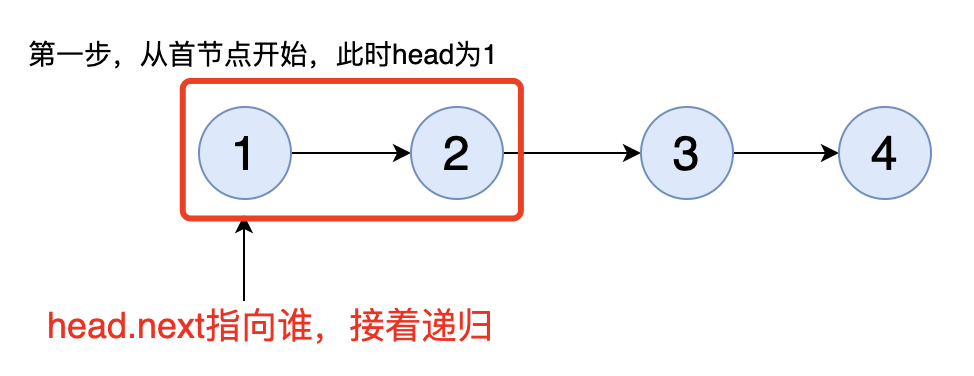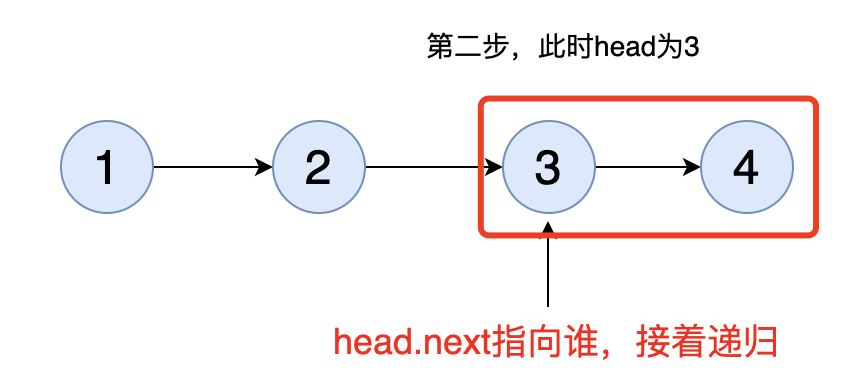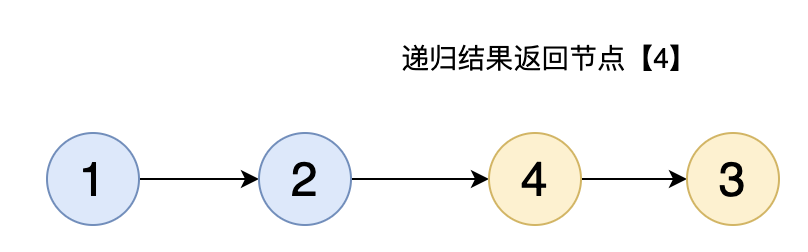##### 3.2 代码分析

``````public ListNode swapPairs(ListNode head) {
if(head == null || head.next == null){
return head;
}
ListNode nextNode = head.next;
head.next = swapPairs(nextNode.next);
nextNode.next = head;
return nextNode;
}
``````
##### 3.3 debug 调试
###### 第一步，节点【1】和节点【2】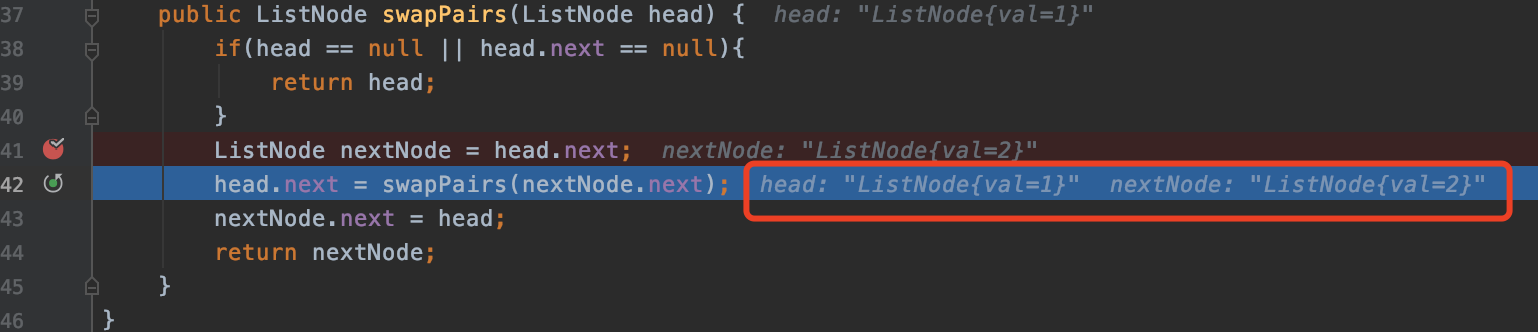###### 第二步，节点【3】和节点【4】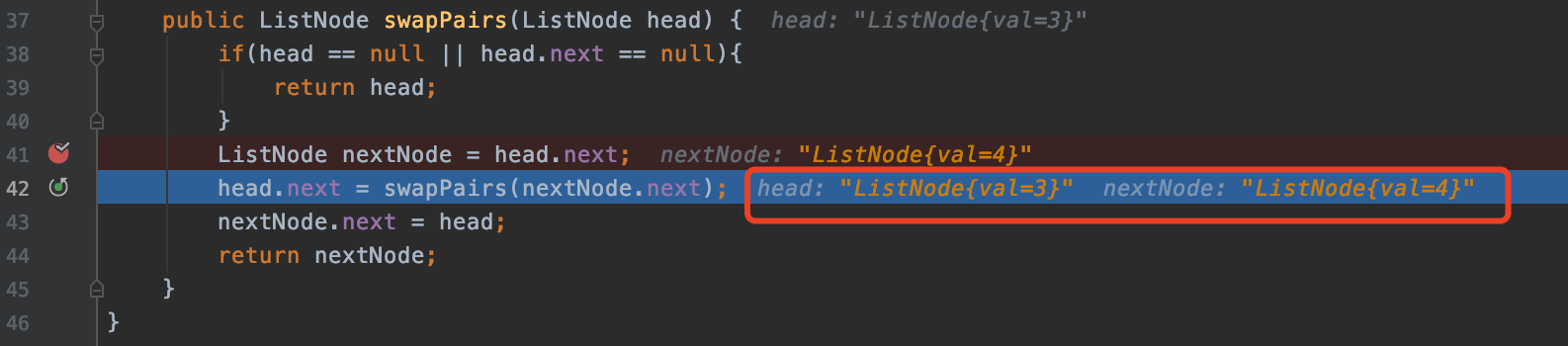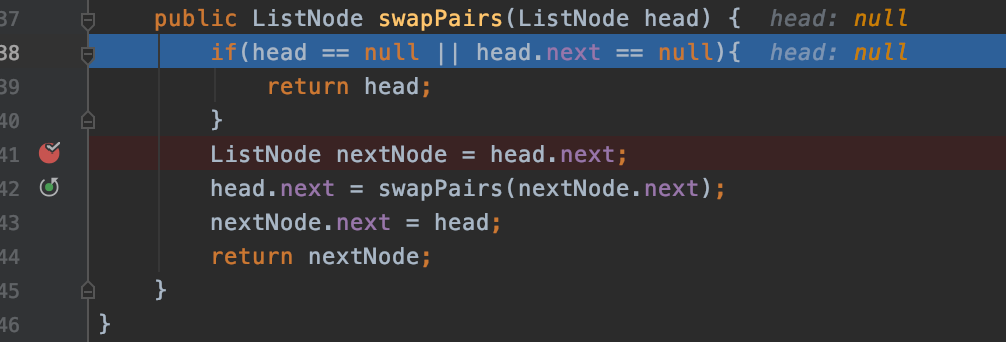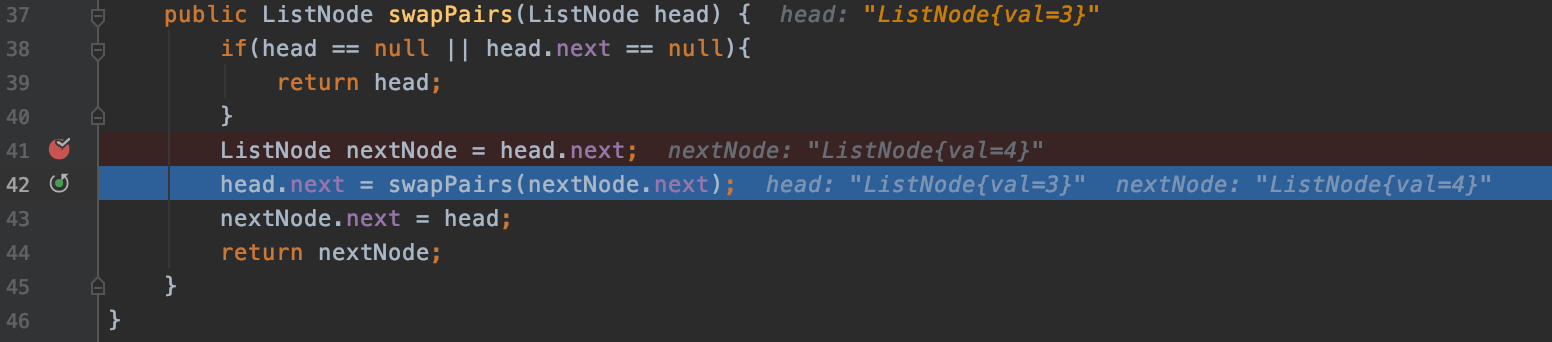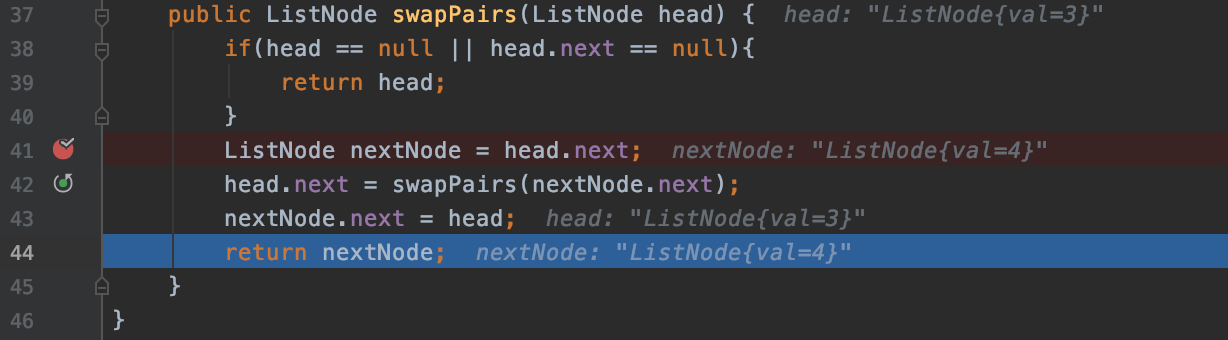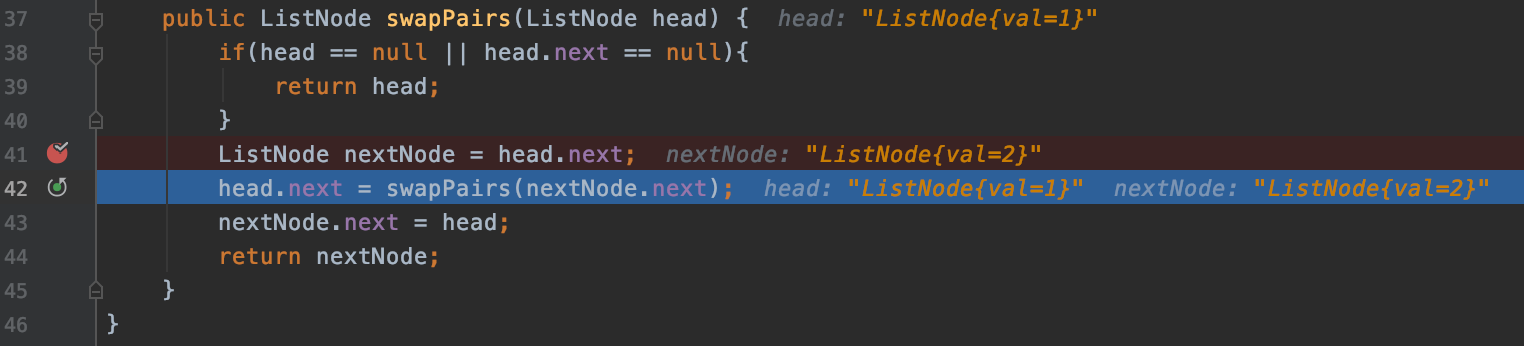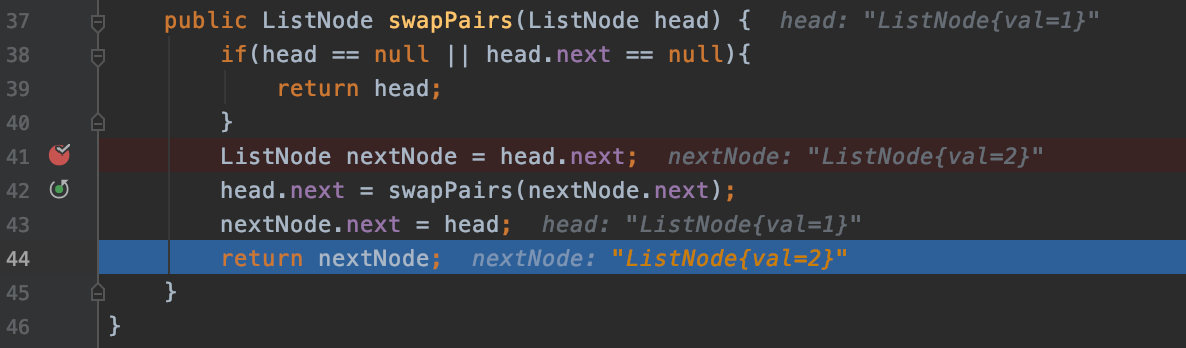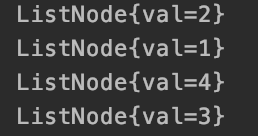###### 3.4 小补充